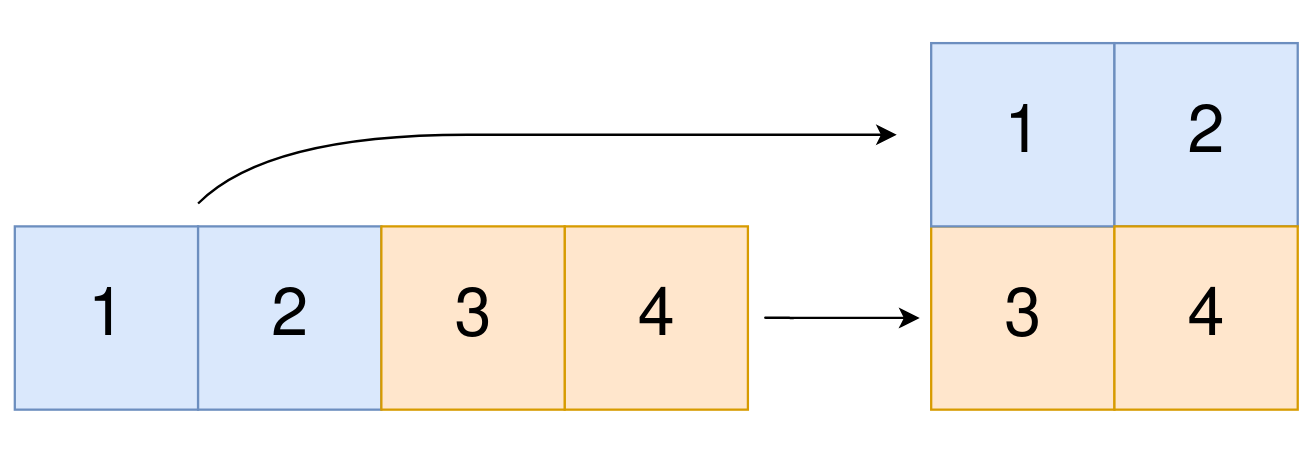Formatted question description: https://leetcode.ca/all/2022.html

# 2022. Convert 1D Array Into 2D Array (Easy)

You are given a 0-indexed 1-dimensional (1D) integer array original, and two integers, m and n. You are tasked with creating a 2-dimensional (2D) array with  m rows and n columns using all the elements from original.

The elements from indices 0 to n - 1 (inclusive) of original should form the first row of the constructed 2D array, the elements from indices n to 2 * n - 1 (inclusive) should form the second row of the constructed 2D array, and so on.

Return an m x n 2D array constructed according to the above procedure, or an empty 2D array if it is impossible.

Example 1:Input: original = [1,2,3,4], m = 2, n = 2
Output: [[1,2],[3,4]]
Explanation:
The constructed 2D array should contain 2 rows and 2 columns.
The first group of n=2 elements in original, [1,2], becomes the first row in the constructed 2D array.
The second group of n=2 elements in original, [3,4], becomes the second row in the constructed 2D array.


Example 2:

Input: original = [1,2,3], m = 1, n = 3
Output: [[1,2,3]]
Explanation:
The constructed 2D array should contain 1 row and 3 columns.
Put all three elements in original into the first row of the constructed 2D array.


Example 3:

Input: original = [1,2], m = 1, n = 1
Output: []
Explanation:
There are 2 elements in original.
It is impossible to fit 2 elements in a 1x1 2D array, so return an empty 2D array.


Example 4:

Input: original = , m = 1, n = 2
Output: []
Explanation:
There is 1 element in original.
It is impossible to make 1 element fill all the spots in a 1x2 2D array, so return an empty 2D array.


Constraints:

• 1 <= original.length <= 5 * 104
• 1 <= original[i] <= 105
• 1 <= m, n <= 4 * 104

Similar Questions:

## Solution 1. Brute Force

// OJ: https://leetcode.com/problems/convert-1d-array-into-2d-array/
// Time: O(K) where K is the length of the original array
// Space: O(1)
class Solution {
public:
vector<vector<int>> construct2DArray(vector<int>& A, int m, int n) {
if (A.size() != m * n) return {};
vector<vector<int>> ans(m, vector<int>(n));
int k = 0;
for (int i = 0; i < m; ++i) {
for (int j = 0; j < n; ++j) {
ans[i][j] = A[k++];
}
}
return ans;
}
};


## Discuss

https://leetcode.com/problems/convert-1d-array-into-2d-array/discuss/1499226/C%2B%2B-Brute-Force